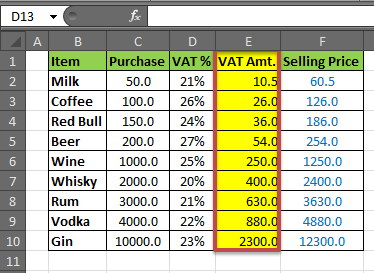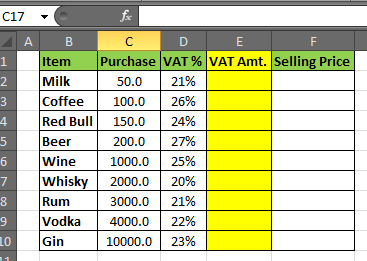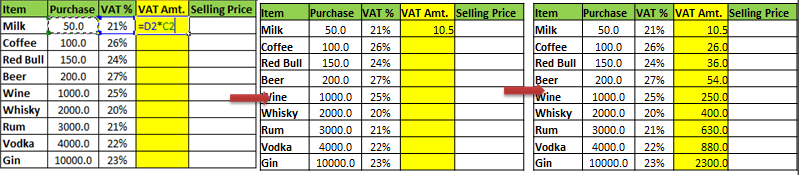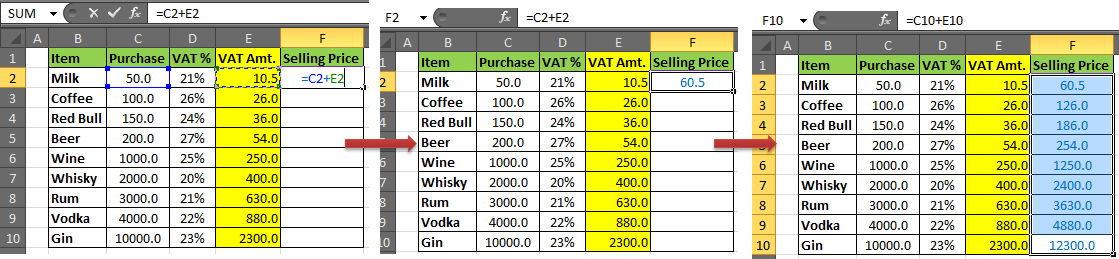# How to Calculate VAT in Excel

In this article you will learn how to calculate VAT in Excel by using a functions? And Which formula we can use to calculate how much VAT has been paid when the only information you have is the total amount?Well, it is quite easy to calculate tax in excel. The VAT (Value Added Tax) is most common type of tax that is applied to goods. Now GST (Good and Services Tax) is applied to almost every kind of goods but for some products like liquors, VAT is still there. You can calculate GST in the same way. After reading this article you will know:

• VAT Calculation in Excel
• How to Calculate the Selling Price of Goods.

Generic Formula of VAT calculation in Excel:

 Purchase Price * VAT%

Generic Formula for Calculating Selling Price :

 Purchase + Taxes

Scenario:

Let’s say we deal with drinks (all kind of). We are assuming that a different VAT% is applied to each product. And that VAT% is given. We need to calculate VAT and Selling Price.

Step 1: Prepare a Table

We prepared below the table in Excel Spreadsheet.Step 2: Calculate the VAT amount

In Cell E2 write this formula and hit enter.

 =D2*C2

You will have your VAT amount calculated in E4 for milk. Drag Down the formula to E10.Note: in Vat% column ‘%’ symbol is necessary. If you don’t want % symbol then you need to write your percentage preceding with “0.” Because excel converts “number%” into “number/100”.

Step 3: Calculate Selling Price:

In Cell F2, write this formula and hit enter.

 =C2+E2

The formula for selling price is ‘Cost+Taxes’ (neglecting profit here). We are assuming that VAT is only tax that is applied to your product. Then the cost is Purchase +VAT amt Taxes.And it is done. Your Vat Calculator in Excel is ready.

You can add Totals in the bottom row or In the rightmost column like most newbies do. But the smart way of showing Totals is on the top row, just above the headers so that it is always visible (if top row is frozen).

Now you know how to calculate vat in Excel. You can use this excel tax formula to create an instant VAT calculator excel spreadsheet. You can reverse the process to create an tax rate formula in excel.

If you liked our blogs, share it with your friends on Facebook. And also you can follow us on Twitterr and Facebook.

We would love to hear from you, do let us know how we can improve, complement or innovate our work and make it better for you. Write us at info@exceltip.com

Popular Articles:

50 Excel Shortcuts to Increase Your Productivity

How to use the VLOOKUP Function in Excel

How to use the COUNTIF function in Excel

How to use the SUMIF Function in Excel

1.TAX AMT. CGST SGST
332600/- 20430.50/- 20430.50/-
18%,12%,5%
Please provide me taxable value for 18%,12%,5% based on above mention figures. Please provide formula for the same.

2.I have gross amount 27864 including VAT 7% how to find net about with vat give excel formula

3.I Have MRP - 17,13,840.00
Excluding Above MRP in 3 Steps - (0) Net Amount (1) SGST 14%, (2) CGST 14% & (3) TCS 1% (sum of 0+1+2+3=MRP)
You have to find 0,1,2,3

Please Help me to find Net Amount, SGST, CGST, TCS from MRP Amount

4.magnetic couple bracelets

I have learn some just right stuff here. Certainly price bookmarking for revisiting. I surprise how a lot effort you put to create this sort of magnificent informative site.

5.ost but I was wondering if you could write a litte more on this subject? I'd be very grateful if you could elaborate a little bit further. Many thanks!

6.7.hi
Please give me formula vat calculation for 5.5%
Like for 14.5% i used to do
Rate = 100
14.5% = 100/1.145= 87.33 Price
100-87.33= 12.67 Vat @ 14.5%
Like wise please give me formula for 5.5% calculation

8.Hi Guys

So i need help...i have used your formulas to calculate the vat and nett selling price. But now i am faced with a dilema.

We are a Guest House right so we purchase many fresh produce which is zero rated. I need to show that on my input tax spreadsheet.

Thank you for your assistance its much appreciated

9.Great article . I was enlightened by the facts ! Does someone know where my assistant could acquire a blank CA FTB 540/540A BK copy to complete ?

10.Dear Admin, thanks for the tips, this is 200% correct formula, I was looking for this formula for a long time. In this tips we just have to put sale price and it calculate vat & net price depending on vat percentage. Thank you so much.

11.VAT/Tax Amount

Select the cell D2, and write the formula to calculate the “VAT/Tax Amount”
=B2*vat
Press Enter
Example, the Vat for Nigeria is 5%
Hence, my formula would translate to;
=B2*5%
or
From the table, the formula should be;
=B2*20%
Press Enter

12.AJIT KUMAR SINGH

Please Give me excel Formula as given below
Basics Rs. 2640000 +Excise 12.5%=+5.5% Vat= Total Tax vale =49335

13.hello what wil be the program codes to calculate the selling price of a produc
sp=bp+vat
vat=tax*bp
tax=16/100

14.anand kondaguli

Tutorial is OK but there are typing errors,
In the example : The correct formula is =B2*100/(100+20)
In the second example: The correct formula is =B2-D2

15.I know the formulas seen on example are right. I have a small business and been going through the arithmetic for a very longtime so as not to make any mistakes that can cost me a lot of money.

My question is, do you use the same formula when you actually give a discount on the selling price before VAT? or you just apply the straight forward deduction of percentage?

I came to this question as when working out a discount with the formula above you can always go back to the selling price by adding the percentage given. If you deduct the percentage strait forward you will find out that there is a small percentage discrepancy that works against the retailer.

•Hi John,

Yes, a retailer will always face a little disadvantage or drawback because of this.

Happy Learning,

16.Subtotal-A: 133.80
Subtotal-B: ( Add 2% on Subtotal-A/ A1) : 136.48
Subtotal-C: 10% Profit ( Add 10% on Subtotal-B) : 150.12
VAT 5.5% of Total 9.12
IT 4% of Total 6.64
Total 165.88

I want how a arrive from 150.12 to 165.88
Paz send me an email

17.Subtotal-A: 133.80
Subtotal-B: ( Add 2% on Subtotal-A/ A1) : 136.48
Subtotal-C: 10% Profit ( Add 10% on Subtotal-B) : 150.12
VAT 5.5% of Total 9.12
IT 4% of Total 6.64
Total 165.88
What is the formula of this rate

I want how a river 150.12 to 165.88

18.PLEASE LET ME KNOW THE FORMULA TO FIND OUT PROFIT AMOUNT :
PROVIDED DETAILS ARE;
COST OF PURCHASE, VAT % AND MRP .

19.how to calculate mrp from selling price

ex-

1000*10%=900
i want from 900 how to arrive 1000

•Hello,

As per the given example, it seems you are keeping 11.11% margin between selling price and MRP. If yes, assuming you have selling price (900) in A2 and you want MRP in B2. Try the following function

=ROUNDUP(A2+A2*11.11%,0)

Please let us know if it works!

Thanks,
Team Excel Forum

20.VAT/Tax Amount
•Select the cell D2, and write the formula to calculate the “VAT/Tax Amount”.
•=B2-B2/(100%+C2)

That formula cannot be correct. B2-B2?

•Hey Tim,

Apply this formula once, I am sure you will get appropriate result.

•Hi there is there not a formula or something like ctrl+V u can use that is easier to work out vat cos i tried the method that was given and does not work for me.

Ta Much

21.Hi,

I raised invoice in Busy Software and this software tax calucation is very good when i put total amount 4000 in this software this software automatically get tax calculation when i print the invoice.
I want know only for is any this calcuation is in excel or not and if yes so plz confirm me what is the formuls.

22.Tip is great. We all know how to add VAT onto the basic price, but I hadn't a clue where to begin doing it the other way around. By the way - VAT is 17.5% in the UK.

•VAT is 20% in the UK, not 17.5%. It has been 20% since the Tories got back into power in 2009. It is one of the first things they did to generate more income for the Government coffers

23."100.00-18% = 82.00 NOT 84.75

Try to find the net price:
A1 100.00 B1 18.00% then try the formula=(100%-b1)*al answer 82.00 "

•You are wrong. you cannot minus the 18% vat/tax directly to the gross amount. 18% is only added into net amount to get the gross price. the tutorial is correct.

100.00 = gross sales
18% = vat

getting net sale price: 100.00/1.18 =84.75
getting the VAT: 100.00/1.18*0.18 =15.25

•Great thank you boss

•Thank you very much NICK .

24."This tip doesn't seem right?
I want to add the tax to the net price"

•to add VAT formula

VAT=ROUND(B2*0.2,2)=540
Total net price including VAT = B2+540=3240

25.please send me some excel formulas

•I don't understand, please send some excel formula

Terms and Conditions of use

The applications/code on this site are distributed as is and without warranties or liability. In no event shall the owner of the copyrights, or the authors of the applications/code be liable for any loss of profit, any problems or any damage resulting from the use or evaluation of the applications/code.